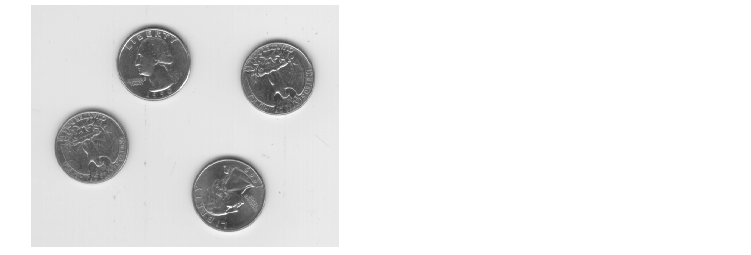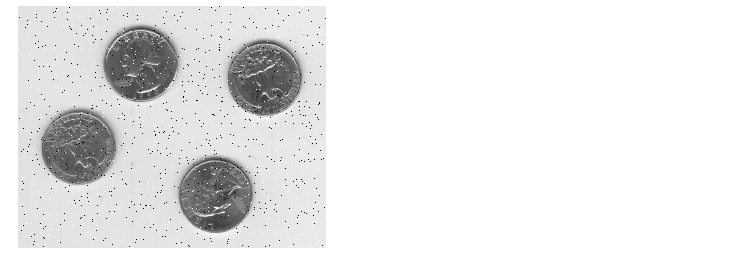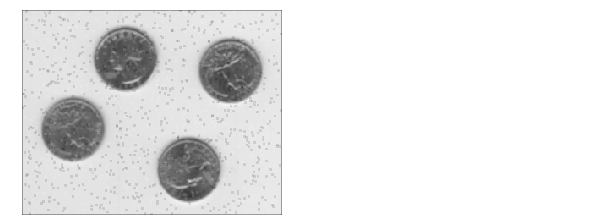Image Processing Toolbox User's GuideUsing Median Filtering

Median filtering is similar to using an averaging filter, in that each output pixel is set to an average of the pixel values in the neighborhood of the corresponding input pixel. However, with median filtering, the value of an output pixel is determined by the median of the neighborhood pixels, rather than the mean. The median is much less sensitive than the mean to extreme values (called outliers). Median filtering is therefore better able to remove these outliers without reducing the sharpness of the image. The `medfilt2` function implements median filtering.

 Note    Median filtering is a specific case of order-statistic filtering, also known as rank filtering. For information about order-statistic filtering, see the reference page for the `ordfilt2` function.

The following example compares using an averaging filter and `medfilt2` to remove salt and pepper noise. This type of noise consists of random pixels' being set to black or white (the extremes of the data range). In both cases the size of the neighborhood used for filtering is 3-by-3.

1. Read in the image and display it.
• ```I = imread('eight.tif');
imshow(I)```
• ```J = imnoise(I,'salt & pepper',0.02);
figure, imshow(J)```
3. Filter the noisy image with an averaging filter and display the results.
• ```K = filter2(fspecial('average',3),J)/255;
figure, imshow(K)```
4. Now use a median filter to filter the noisy image and display the results. Notice that `medfilt2` does a better job of removing noise, with less blurring of edges.
• ```L = medfilt2(J,[3 3]);
figure, imshow(K)
figure, imshow(L)```Using Linear Filtering Using Adaptive Filtering